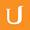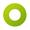## 04-02 Analyzing Alice's Algorithm

• 0:00 - 0:09
And the correct answer here is O(2n*n²) and the reason why this is correct is as follows--
• 0:09 - 0:16
so the first line here takes constant amount of times so that's not going to be relevant.
• 0:16 - 0:26
Now, this loop here lines 2 to 5 are executed 2n*, so the question is each time this interloop here
• 0:26 - 0:29
lines 3 to 5 is executed, how much time does that take.
• 0:29 - 0:36
So checking of the assignment is valid, takes O(2n²*), then if the assignment is valid,
• 0:36 - 0:42
we need an additional O(n)+O(1), so an additional O(n) time because this here is constant,
• 0:42 - 0:44
so we can ignore it.
• 0:44 - 0:52
So the maximum time that lines 3 to 5 take is O(n²)+O(n)+O(1),
• 0:52 - 1:01
so the largest growing term here is O(n²)--so the interloop takes O(n²*) and that is executed 2n*.
• 1:01 - 1:08
So that's why the running time of Alice's algorithm is O(2n*n²),
• 1:08 - 1:12
and the good thing is that because we have our own notation, we could do this analysis
• 1:12 - 1:16
without actually concretely stating how all of these algorithm is programmed.
• 1:16 -
So as I hoped you see, it's quite a useful notation to have.
Title:
04-02 Analyzing Alice's Algorithm
Team:Udacity
Project:
CS313 - Theoretical Computer Science
Duration:
01:21Amara Bot added a translation

• Amara Bot